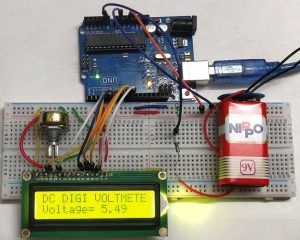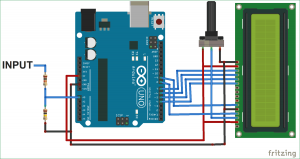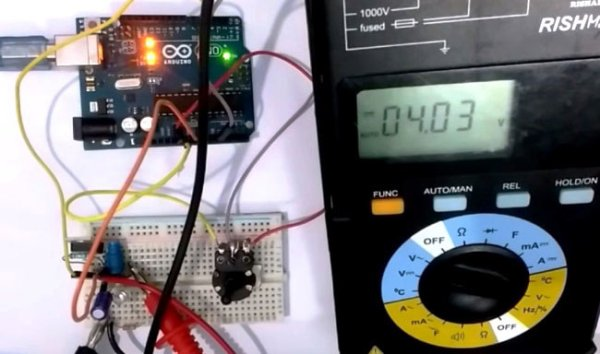# Simple Arduino Digital Voltmeter

With a simple knowledge of Arduino and Voltage Divider Circuit, we can turn the Arduino into Digital Voltmeter and can measure the input voltage using Arduino and a 16×2 LCD display.The voltage measured is displayed on the 16×2 Liquid Crystal Display (LCD). We have also displayed the voltage in Serial Monitor of Arduino IDE and confirmed the measured voltage using Multimeter.

### Hardware Required:

• Arduino uno
• 16×2 LCD (Liquid Crystal Display)
• 100 k ohm resistor
• 10 k ohm resistor
• 10 k ohm potentiometer
• jumper wires

### Voltage Divider Circuit:

Before entering into this Arduino Voltmeter circuit, lets discuss about the Voltage Divider Circuit.

Voltage divider is a resistive circuit and is shown in figure. In this resistive network we have two resistors. As shown in figure, R1 and R2 which are of 10k and 100k ohm. The midpoint of branch is taken to measurement as a anolog input to the Arduino. The voltage drop across R2 is called Vout , that’s the divided voltage of our circuit.

Formulae:

Using the known value (two resistor values R1, R2, and the input voltage), we can substitute in the equation below to calculate the output voltage.

READ  Speech Recognition with Arduino and BitVoicer Server

Vout = Vin (R2/R1+R2)

This equation states that the output voltage is directly proportional to the input voltage and the ratio of R1 and R2.

By applying this equation in the Arduino code the input voltage can be easily derived. Arduino can only measure the DC input voltage of +55v, In other words, when measuring 55V, the Arduino analog pin will be at its maximum voltage of 5V so it is safe to measure within this limit. Here the resistors R2 and R1 value is set to 100000 and 10000 i.e. in the ratio of 100:10.### Circuit Diagram and Connections:

Connection for this Arduino Digital Voltmeter is simple and shown in the circuit diagram below:

Pin DB4, DB5, DB6, DB7, RS and EN of LCD are directly conneted to Pin D4,  D5, D6, D7, D8, D9 of Arduino Uno

The Center point of two resistors R1 and R2, which makes the voltage divider circuit, is connected to Arduino Pin A0. While the other 2 ends are connected to the input volt (voltage to be measured) and gnd.

Read more: Simple Arduino Digital Voltmeter